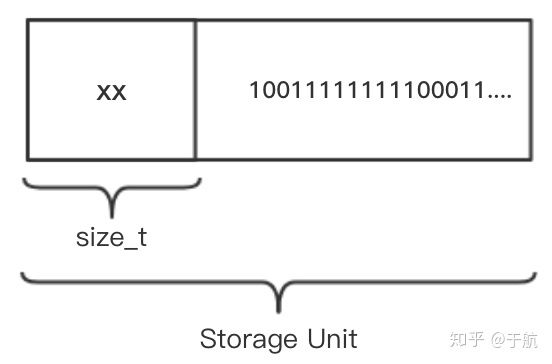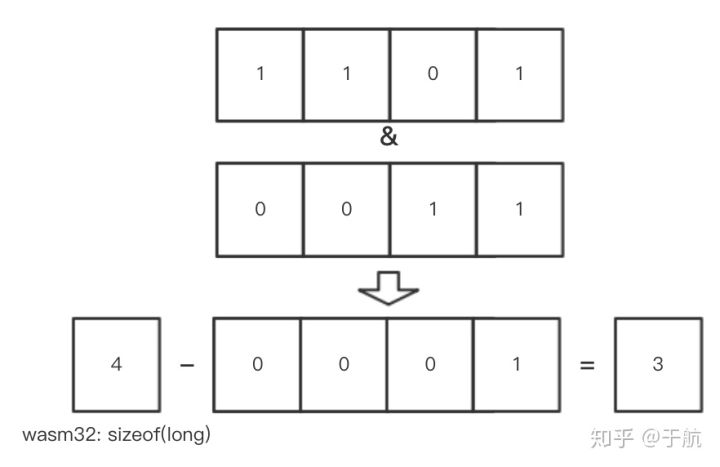X

# Redis 源码之内存管理 - zmalloc / zfree

``````void *zmalloc(size_t size) {
// 分配内存；
void *ptr = malloc(size + PREFIX_SIZE);
// 分配失败抛出异常；
if (!ptr) zmalloc_oom_handler(size);
// 系统是否可以使用”malloc_size“函数？
#ifdef HAVE_MALLOC_SIZE
update_zmalloc_stat_alloc(zmalloc_size(ptr));
return ptr;
#else
// 在数据域保存分配数据的实际大小；
*((size_t*)ptr) = size;
// 计算对齐后的内存使用大小，并更新”used_memory“变量；
update_zmalloc_stat_alloc(size + PREFIX_SIZE);
// 返回数据体的初始位置；
return (char*)ptr + PREFIX_SIZE;
#endif
}
````````````#define update_zmalloc_stat_alloc(__n) do {
size_t _n = (__n);
// 手动内存补齐；
if (_n&(sizeof(long)-1)) _n += sizeof(long)-(_n&(sizeof(long)-1));
atomicIncr(used_memory, __n);
} while(0)
``````

``````void zfree(void *ptr) {
#ifndef HAVE_MALLOC_SIZE
void *realptr;
size_t oldsize;
#endif

if (ptr == NULL) return;
#ifdef HAVE_MALLOC_SIZE
update_zmalloc_stat_free(zmalloc_size(ptr));
free(ptr);
#else
realptr = (char*)ptr-PREFIX_SIZE;
oldsize = *((size_t*)realptr);
update_zmalloc_stat_free(oldsize+PREFIX_SIZE);
free(realptr);
#endif
}
``````

``````#define update_zmalloc_stat_free(__n) do { \
size_t _n = (__n); \
if (_n&(sizeof(long)-1)) _n += sizeof(long)-(_n&(sizeof(long)-1)); \
atomicDecr(used_memory,__n); \
} while(0)
``````